## 2.4.2 Electronic Band Structure of SW-CNTs

The electronic structure of a SW-CNT can be obtained from that of graphene. Assuming periodic boundary conditions in the circumferential direction characterized by the chiral vector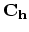, the wave vector associated with thedirection becomes quantized, while the wave-vector associated with the direction of the translational vector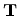(along the CNT axis) remains continuous for a CNT of infinite length. Thus the energy bands consist of a set of one-dimensional energy dispersion relations which are cross sections of those of graphene. Expressions for the reciprocal lattice vectors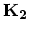along the CNT axis, (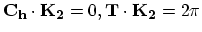), and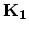in the circumferential direction, (), are given by (see Table 2.2)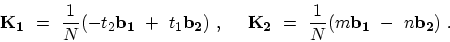(2.5)

The one-dimensional energy dispersion relations of a SW-CNT can be written as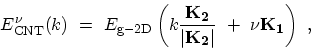(2.6)

where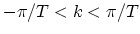is a one-dimensional wave-vector along the CNT axis and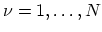. The periodic boundary condition for a CNT gives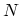discrete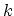values in the circumferential direction. The N pairs of energy dispersion curves given by (2.6) correspond to the cross sections of the two-dimensional energy dispersion surface of graphene. In Fig. 2.7 several cutting lines near one of the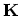points are shown. The separation between two adjacent lines and the length of the cutting lines are given by the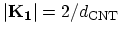and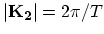, respectively. If the cutting line passes through apoint of the two-dimensional BRILLOUIN zone (Fig. 2.7-a), where the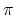and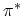energy bands of graphene are degenerate by symmetry, then the one-dimensional energy bands have a zero energy gap. When the-point is located between to cutting lines,is always located in a position one-third of the distance between two adjacentlines (Fig. 2.7-b)  and thus a semiconducting CNT with a finite energy gap is formed. If for a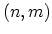CNT,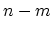is exactly divisible by 3 the CNT is metallic. CNTs with residuals 1 and 2 of the divisionby 3 are semiconducting.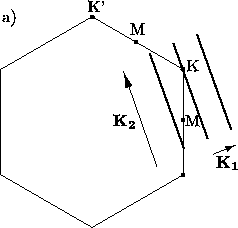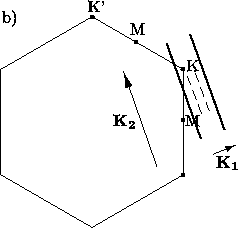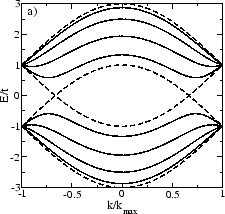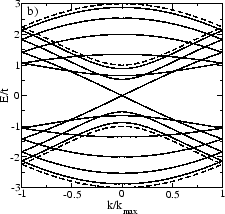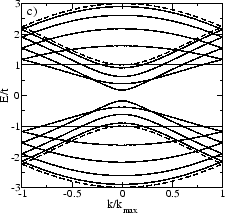Figure 2.8 shows the energy dispersion relations for the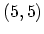armchair, the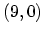zigzag, and the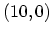zigzag CNTs. In general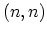armchair CNTs yield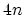energy subbands with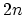conduction andvalence bands. Of thesebands, two are non-degenerate and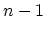are doubly degenerate. The degeneracy comes from the two subbands with the same energy dispersion, but different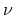-values. All armchair CNTs have a band degeneracy between the highest valence and the lowest conduction band (Fig. 2.8-a). In zigzag CNTs the lowest conduction and the highest valence bands are doubly degenerate (Fig. 2.8-b and Fig. 2.8-c).

In armchair and zigzag CNTs the bands are symmetric with respect to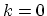. Since the band of an armchair CNT has a minimum at point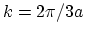, it has a mirror minimum at point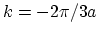and therefore two equivalent valleys are present around the point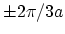. The bands of zigzag and chiral CNTs can have at most one valley (Fig. 2.8-b and Fig. 2.8-c).

In armchair CNTs the bands cross the FERMI level at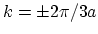. Thus, all they are expected to exhibit metallic conduction . There is no energy gap for theCNT at, whereas theCNT indeed shows an energy gap.

Electrical conduction is determined by states around the FERMI energy. Therefore, it is useful to develop an approximate relation that describes the dispersion relations in the regions around the FERMI energy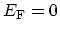. This can be done by replacing the expression for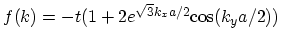in (2.3) with a TAYLOR expansion around the point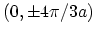where the energy gap is zero and. It is straightforward to show that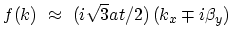, with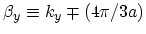. The corresponding energy dispersion relation can be written as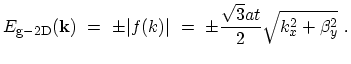(2.7)

The energy bands for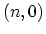zigzag CNTs can be obtained by imposing the periodic boundary conditions, which define the number of allowed wave-vectors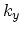in the circumferential direction as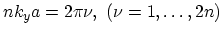. This yields the one-dimensional dispersion relations for thestates of thezigzag CNT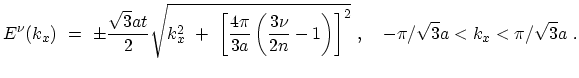(2.8)

Therefore, the energy gap for subbandcan be written as difference between the energies of the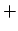and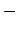branches at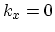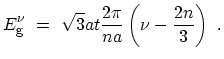(2.9)

The energy gap has a minimum value of zero corresponding to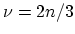. If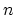is not a multiple of three the minimum value ofis equal to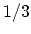. This means that the minimum energy gap is then given by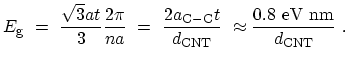(2.10)

where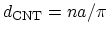is the diameter of the CNT in nano-meters. Based on (2.8) and (2.9), the DOS for semiconducting zigzag CNTs is given by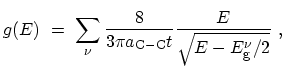(2.11)

which is an approximation valid as long as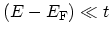. VAN HOVE singularities in the DOS appearing at both the energy minima and maxima of the bands (see Fig. 2.9) are important for determining various solid-state properties of CNTs [35,36]

For all metallic CNTs, independent of their diameter and chirality, due to the nearly linear dispersion relations around the FERMI energy the density of states (DOS) per unit length along the CNT axis is a constant given by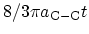.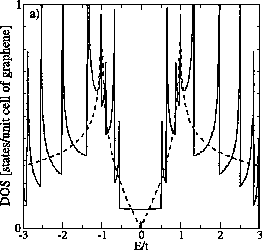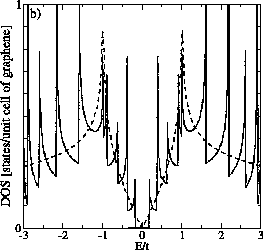M. Pourfath: Numerical Study of Quantum Transport in Carbon Nanotube-Based Transistors